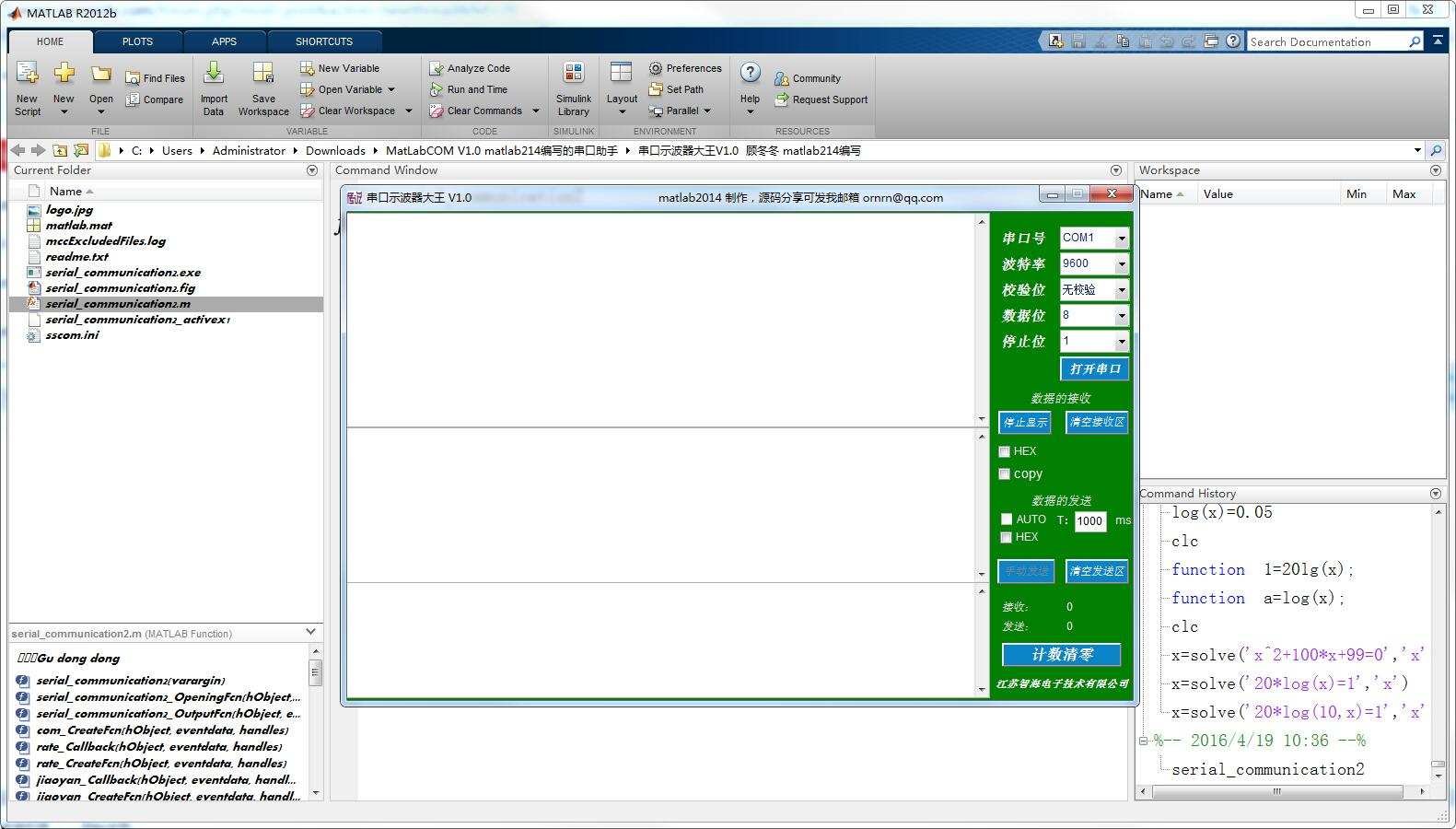# [经验] MATLAB串口助手小程序分享！

2019-8-2 03:06:37158 matlab 欧拉角
 这里先分享一下，我用matlab写的一个串口助手小程序 下面是21IC.COM  "gaoyang9992006" 的帖子分享： 开始做四轴了，一步一步来，东西实在很多，比较杂。先做matlab上位机，主要用来做数据分析，等板子到了可以写飞控的程序了，从底层一层一层开始写。。希望能好好的完成它。。。关于matlab上位机，首先做个姿态显示，然后等板子来了，把板子底层程序写好后，加上matlab的串口接收部分，基本的环境就算搭建好了。。。。         这个代码写了一天，写到最后出现戏剧性的一幕，实在是太恶心了哈。。开始自己的想法就是通过输入pitch roll yaw三个欧拉角，然后在空间中现实飞机的姿态，为了学习matlab翻了matlab的书，还看了线性代数，为了画这个姿态图，看了高中的立体解析几何，向量运算等。。。都是泪啊，说回正题，首先计算xOy平面中的转动，也就是yaw轴，这个相对比较简单，让三角形的三个点分别在图中的大圆和小圆上，如图所示： yaw解决了之后就需要解决pitch了，就是俯仰角，约定是以坐标的（0 0 0）点进行旋转的，也是两个圆的圆心，所以算pitch只需要在xOz平面内计算，通过sin（pitch）可以算出来A B C三个点在Z轴上的坐标了，这里需要注意下，A点变换后，相对应的X轴变化是cos(pitch),y轴也是，算到这里会发现一个问题，用matlab算B C连个点的时候，只需算B或者C，解出来是有两个解的，一个B一个C，B和C必须分辨清楚，否则在计算roll的时候因为 B C没有分清楚会导致roll旋转方向不确定，后面再说B C怎么分辨。         接下来是计算 roll了，需要计算B 点和C点在Z轴上的坐标，因为我们是绕着（0 0 0）转的，而不是绕着BC的终点转，所以无法通过BC的长度乘以sin（roll）计算，所以通过圆心做一条直线与BC平行，假设与AC交与F点， %          A %       E  O  F %   B      D     C 无论pitch和yaw怎么转，OF都是在xOy平面的，方便计算，通过sin（roll）*OF的长度就可以得到F在Z轴的变化，从而通过等比可以的到C在Z轴的变化，B点变化和C是一样的，方向相反，之后将B C的坐标在xOy平面做cos（roll）缩放就可以的到最终的三角形的三个坐标了。        接着讲BC的分辨问题，想来想去只想到一个比较简单的方法，我们算出来BC并不知道哪个是B，哪个是C，不过我们可以制定一个B‘ 点，那就是我们取一个DB方向的方向向量n，跟随三角形旋转，让它始终指向定义的DB方向，然后可以计算OB OC分别和向量n的内积，因为n与OB为锐角，与OB为钝角，so，n 与OB点乘为负数，与OC点乘为正数，从而区分出B点和C点 。         上面想法看起来不错，但是怎么让向量n随着yaw角转动呢，灵机一闪，线性代数书的矩阵里面有个旋转矩阵啊，立马拿过来验证，发现可以很好的运行，然后想到一个问题，如果某种情况三角形roll为90度，DB的分量在xOy平面为0，这个方法就无效了啊（其实这个问题应该不会出现，因为我们是线计算yaw 然后计算pitch，在计算pitch的时候分辨BC亮点，压根就还没开始计算roll），那用三维旋转矩阵就可以解决这个问题啊，嗯嗯，又灵机一闪，之前看过捷联惯性导航书上讲了方向旋转矩阵啊，应该可以用。把方向余弦拿过来计算一下，和用xOy平面的旋转举证效果一样，到此忽然想到一个非常十分**的事情，妈蛋，三角形三个点全部用这个方向余弦矩阵旋转就可以了啊，立马改程序，不到十分钟就改完了，程序运行良好，都是泪。。。。。。不过自己的算法不能半途而废啊，后面还是把自己的算法完成，并且也可以很好的运行。。。不过因为用了matlab的符号运算，速度和用方向余弦计算比起来慢很多，后面还是用方向余弦算吧。。。。。。。 下面贴代码： [Golang] 纯文本查看 复制代码%% %2014.7.19 由 sky.zhou 编写 function DrawAttitude(pitch,roll,yaw)%%%用于显示飞机姿态，输入为pitch，roll，yaw。 %自己的2B算法算的太慢了，我勒个去。。。还是用方向余弦吧 mode = 2       %标记用那种方法进行计算，1：表示用自己写的2B算法进行计算，2表示用方向余弦矩阵进行计算    %pitch = 60; %roll = 45; %yaw = 35; r1 =3;        %大圆半径 r2 = 0.618*r1;    %小圆半径   IF mode == 2     pitch = -pitch;   %角度定义不一样，改一下     roll = -roll;     %角度定义方式不一样，自己习惯改就好，看你希望是以怎样的方向转 end dc = [cosd(yaw)*cosd(pitch)-sind(yaw)*sind(roll)*sind(pitch)   sind(yaw)*cosd(pitch)+cosd(yaw)*sind(roll)*sind(pitch)   cosd(roll)*(-sind(pitch));       sind(yaw)*(-cosd(roll))                          cosd(yaw)*cosd(roll)                            sind(roll)        ;       cosd(yaw)*sind(pitch)+sind(yaw)*sind(roll)*cosd(pitch)   sind(yaw)*sind(pitch)-cosd(yaw)*sind(roll)*cosd(pitch)      cosd(roll)*cosd(pitch) ] %三角形规约：A为定点，B C为两边的角，具体方位如下 %       A %     B   C t_fpa = 35;      %三角形定点角度设置为40度，fpa On behalf of Fixed point angle t_b = (180 - t_fpa) / 2; t_c = t_b;   if t_fpa > asind((r2/r1))*2     t_fpa = asind((r2/r1))*2 end   %xd,yd,zd存放真是数值，与符号xyz区分开来 %约定 xd yd zd 第 1 2 3 4位分别代表三角形ABC的 A、B、A、C坐标 if mode == 2    xd=[3 -1.2735;3 -1.2735];    yd=[0  1.3474;0  -1.3474];    zd=[0 0;0 0];    %上面几个初始化的点是根据 定义的。    %pitch = 0;    %roll = 0;    %yaw = 0;    %r1 =3;        %大圆半径    %r2 = 0.618*r1;    %小圆半径 else    xd=[];    yd=[];    zd=[];    tempA =[];     %保存中间计算角度，目前之用来保存角BOA end    temp = []; if mode == 2    temp = [xd(1,1) yd(1,1) zd(1,1);            xd(1,2) yd(1,2) zd(1,2);            xd(2,2) yd(2,2) zd(2,2)];    temp = temp*dc;    xd = [temp(1:2,1)';temp(1,1),temp(3,1)]    yd = [temp(1:2,2)';temp(1,2),temp(3,2)]    zd = [temp(1:2,3)';temp(1,3),temp(3,3)]    %到此位置，方向余弦矩阵已经计算完毕，可以直接用后面的函数进行显示 end   if mode == 1      %执行自己的2B算法 %xs ys zs分别问记录方程的解 xs 为sysm缩写 syms x y z r xs ys zs;                                      %x y z 惯性坐标系中三个正交基，r为xOy平面中的大圆和小圆半径 %定义各点的坐标符号参数 syms xa ya za xb yb zb za zb zc ;   %% c1 = sym('x^2+y^2 = r^2');                         %大圆方程 c1 = subs(c1,'r',r1)                               %换成实际数值   c2 = sym('x^2+y^2 = r^2');                         %校园方程，可以表达为：c2 = 'x^2+y^2 = r^2'，效果是一样的 c2 = subs(c2,'r',r2)   l1 = sym('cosd(yaw)*y=sind(yaw)*x') %l1 = sym('y=tand(yaw)*x')                         %不用这个公式是因为这个公式有零点，90和-90无法使用 %l1 = subs(l1, 'yaw', yaw)                         %换成实际数值,这里不要转成实际数值，为了方便subs的运算 %% [xs ys] = solve(c1,l1,'x','y')                     %注意，这里算出来的xd yd是符号变量，matlab自动转换了，下面重新对其赋值，可以变回数值变量    %双百分号还可以类似于分类的作用，挺好。 temp = subs([xs;ys])   %% %计算A点坐标 if yaw > -90 && yaw < 90                             %判断角度的范围，用来选择在坐标中三角形的顶点是正还是负     %这个可能有点难理解，角度确定了，就可以知道焦点在x轴的正负，从前两个数值中取对应的X解后，然后取对应的Y的解     temp = temp([temp(1:2)>0;temp(1:2)>0]) elseif yaw == -90     temp = [ 0 ;temp(temp<0)] elseif yaw == 90     temp = [ 0 ;temp(temp>0)] else     temp = temp([temp(1:2)<0;temp(1:2)<0]) end   %得到在XOY平面中三角形定点的第一个解 xd = [xd temp(1)] yd = [yd temp(2)]   %% %计算B点坐标   %temp计算出来表示的是 AB段的长度， %       A %       O %    B  D  C %其中 sind(t_b/2)*r2 表示的是OD段的长度，cosd(t_b/2)*r2是BD段的长度， %temp计算的最终结果是AB的长度 %利用三角形边与对面角正弦成比例进行运算 %   AB     BC          A0                B0 % ----- = -----       -----     =   --------- % sin(C)  sin(A)     sin(角ABO)      sin(角OAB)（ps:A的一半） %   可以求出角ABO，然后通过内角和可以求出角AOB %   AB                BO          % -----       =     --------      可以求出AB长度，简化代码如下     % sin(角AOB)        sin(角OAB)     % (180 - asind((r1/r2)*sind(t_fpa/2)) - (t_fpa/2)) 为角BOA的大小 tempA = sym('(180 - asind((r1/r2)*sind(t_fpa/2)) - (t_fpa/2))'); temp = sym('(r2/sind(t_fpa/2))*sind(tempA)'); tempA = subs(tempA); temp = subs(temp);     %temp = subs(sym('sqrt(((sind(t_b/2)*r2)+r1)^2 + (cosd(t_b/2)*r2)^2)'));   %假设 符号 xa ya 为 A点的坐标,x,y为要求的B点坐标 temp = subs(sym('(x-xa)^2 + (y-ya)^2 = temp^2'),'temp',temp); %将xa和ya换成数值xa和ya，嵌套换的 temp = subs(subs(temp,'xa',xd(1)),'ya',yd(1)) [xs ys] = solve(temp,c2,'x','y')       %通过下面的计算就已经可以得到 B C的坐标了 temp = subs([xs;ys])   %下面需要做的是区别哪个点是A，哪个点是B。 %% %    下面是在xOy平面内的旋转 %    B   %    D  O  A       yaw=0度的时候三角型在X0Y平面的方位，其中水平位置为x轴竖直方向为Y轴 %    C %    取一个与DB方向一样的方向向量n（0，1） %    用旋转矩阵让它跟三角形同步旋转 %    因为n与OB为锐角，与OB为钝角，so，n与OB点乘为负数，与OC点乘为正数，从而区分出B点和C点 %% %    为了避免roll为90度的时候按照之前的定义方向向量n=（0，0），区分不出来B和C点，所以用方向余弦矩阵进行计算 %方向余弦矩阵定义 %dc = [cosd(yaw)*cosd(pitch)-sind(yaw)*sind(roll)*sind(pitch)   sind(yaw)*cosd(pitch)+cosd(yaw)*sind(roll)*sind(pitch)   cosd(roll)*(-sind(pitch)); %      sind(yaw)*(-cosd(roll))                          cosd(yaw)*cosd(roll)                            sind(roll)        ; %      cosd(yaw)*sind(pitch)+sind(yaw)*sind(roll)*cosd(pitch)   sind(yaw)*sind(pitch)-cosd(yaw)*sind(roll)*cosd(pitch)      cosd(roll)*cosd(pitch) ] %%算到这里的时候我发现只要在xOy平面内将三角形的初始化坐标ABC三个点输入后，用方向余弦矩阵算就可以了，然后花了10分钟不到的时间就实现了 %不过这里还是决定把这个方法写完。。。都是泪。。。。。。。。。。。。。。。。。 %% n  = [0  1  0]                                         %方向向量 n  = n*dc                                               %对方向向量进行旋转 %约定 xd yd zd 第 1 2 3 4位分别代表三角形ABC的 A、B、A、C坐标 n = n*[temp(1);temp(3);0] if n > 0       %说明夹角是锐角，该角是B点     xd = [ xd temp(1) xd temp(2)]     yd = [ yd temp(3) yd temp(4)] else     xd = [ xd temp(2) xd temp(1)]     yd = [ yd temp(4) yd temp(3)] end   %处理成变成矩阵形式 xd = [xd(1:2);xd(3:4)] yd = [yd(1:2);yd(3:4)]   %当存在pitch角度的时候，X坐标做相印调整 xd = xd.*cosd(pitch) yd = yd.*cosd(pitch)     %% %约定 xd yd zd 第 1 2 3 4位分别代表三角形ABC的 A、B、A、C坐标 %计算z中A的坐标，其中B和C是相等的 zd = [zd sind(pitch)*r1]   %下面OD的长度，然后可以计算出B和C在Z轴上的坐标,也就是D点的坐标 od = (sind(tempA - 90)*r2) %zd = [zd temp;zd temp]   %计算roll状态下B和C的坐标 %          A %       E  O  F %     B    D    C %    先计算在roll下OF的长度，然后算F在Z轴的高度，然后等比后算B和C在Z轴的高度 %下面计算OF的长度 l2 = tand(t_fpa/2)*r1 %下面计算F在Z轴上的变化高度 l2 = sind(roll)*l2 %下面计算C点在Z轴上的变化高度,通过相似三角形计算 l2 = l2*(r1+od)/r1   zd = [zd -l2;zd l2] %x,y轴根据picth角度缩放 yd(:,2) = yd(:,2).*cosd(roll) xd(:,2) = xd(:,2).*cosd(roll)   %额。。这方法写的心力交瘁。。。。。。。还是方向余弦好。。。四元素再学。。。。。。     end suRF(xd,yd,zd) axis([-3 3 -3 3 -3 3]) xlabel('X') ylabel('Y') zlabel('Z') text(xd(1,1),yd(1,1),zd(1,1),'A点') text(xd(1,2),yd(1,2),zd(1,2),'B点') text(xd(2,2),yd(2,2),zd(2,2),'C点') %% %测试用圆 hold on alpha=0:pi/20:2*pi; x=r1*cos(alpha); y=r1*sin(alpha); plot(x,y);   hold on x=r2*cos(alpha); y=r2*sin(alpha); plot(x,y);   hold off endMatLabCOM V1.0 matlab214编写的串口助手.rar (7.18 MB )

## 18 条评论

 这帖子一般都喜欢下载不说话，你是第一个回复的哈哈
 厉害啊！学习一下。谢谢楼主。

## 站长推荐/10•最新主题
•推荐主题
•热门主题
•我的帖子
-

-

-

-

-

-

-

-

-

-